• Picking the right learning rate at different iterations helps model to converge quickly.
• The 1cycle policy gives very fast results when training complex models. It follows the Cyclical Learning Rate (CLR) to obtain faster training time with regularization effect but with a slight modification.
• Specifically, it uses one cycle that is smaller than the total number of iterations/epochs and allow learning rate to decrease several orders of magnitude less than the initial learning rate for the remaining iterations (i.e. last few iterations).
• Philosophy behind CLR is a combination of curriculum learning and simulated annealing.
• For certain hyper-parameter values, using very large learning rates with CLR method can speed up training by as much as an order of magnitude. This phenomenon is what Leslie Smith described as Super-Convergence.

## Varying Learning Rates

In the paper Super-Convergence: Very Fast Training of Neural Networks Using Large Learning Rates, Leslie Smith recommends a cycle with 2 steps of equal lengths, first step going from lower learning rate to higher before second step to back to minimum. Note that the increase and decrease are changing linearly. The maximum should be picked using the Learning Rate Finder and the minimum can be set to a factor of 3 or 4, whereas the lowest should be reduced by a factor of 10 of the minimum. Lastly, the length of the cycle should be less than total number of epochs, leaving the last epochs to a learning rate even lower than the minimum (by several orders of magnitude.

Leslie Smith also observed that in the middle of the cycle, high learning rates will act as regularization method, keeping the network from over-fitting. This is because large learning rates add noise/regularization in the middle of training and higher levels of noise lead SGD to solutions with better generations.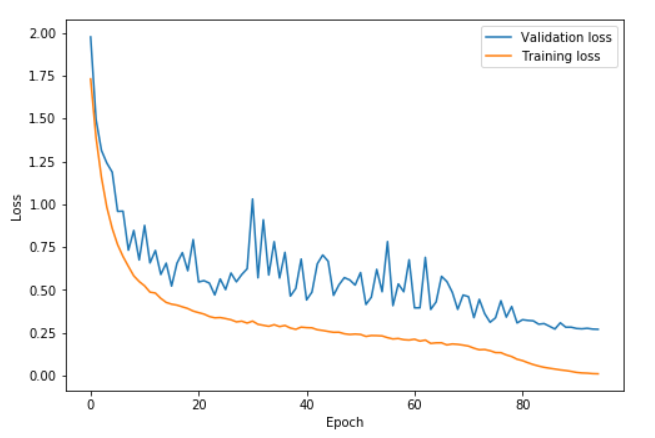Fig. 2 – In the last epochs, lower learning rate (beyond the minimum) helps to achieve lower loss

In Fig. 2, learning rate rose from 0.08 to 0.8 between epochs 0 and 41, and got back to 0.08 between epochs 41 to 82, before going to 0.0008 in the last few epochs. We can see how validation loss is more volatile during the high learning rate part of the cycle (epoch 20 to 60) but the difference between the training loss and validation loss doesn’t increase. Over-fitting starts at the end of the cycle, where learning rate goes really lower (annihilated).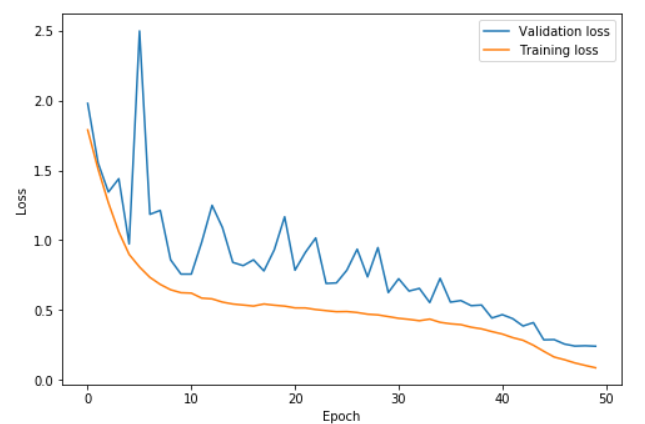Fig 3. – Higher learning rate helps to learn faster and prevent overfitting

In Fig. 3, learning rate rose faster from 0.15 to 3 between epoch 0 and 22.5 and got back to 0.15 between 22.5 and 45, before going to 0.0015 in the last few epochs. Such a high learning rates help to learn faster and prevent over-fitting. Comparing it against Fig. 2, we manage to reach a lower loss in lesser epochs.

Notice the difference between validation loss and training loss stays extremely low until we bring the learning rate even lower (at the last few epochs), then we see a the difference get higher.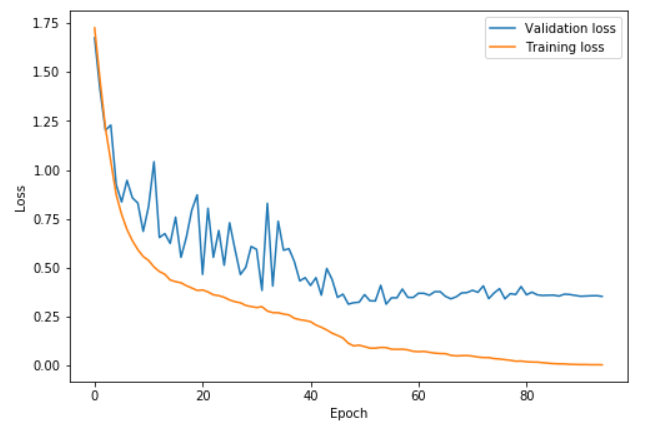Fig. 4 – Smaller cycle followed by longer annihilation results in bigger over-fitting

In Fig. 4, we use a smaller cycle and a longer annihilation results in over-fitting. As seen from epoch ~45, there is large difference between training and validation loss, indicating over-fitting. The difference between training and test accuracy is also known as generalization gap.

## Super-ConvergenceFig. 5 – In the paper “Super-Convergence”, Leslie showed the improvement in validation accuracy by comparing standard learning rate policy and 1cycle policy.

In Fig. 5, Leslie presented the improvement in validation accuracy by comparing the standard learning rate policy and 1cycle policy. The typical, standard learning rate policy, or a piecewise-constant training regime refers to the practice of using a global learning rate (e.g. 0.1) for many epochs until the test accuracy plateaus, and then continuing to train with a learning rate decreased by a factor of 0.1. This process of reducing learning rate is repeated 2 to 3 times in the entire training.

We can see that by using 1cycle policy, the deep neural networks can be trained much faster than using the standard learning rate policy.

## Cyclical Momentum

In order to use such large learning rates as proposed by the 1cycle learning rate schedule, it was necessary to reduce the value for weight decay. With that, Leslie discovered that decreasing the momentum led to better results. The intuition is that we want SGD to quickly go in the directions to find a flatter area, so new gradients need to be given more weights than the old. Picking the values for momentum between 0.85 and 0.95, and starting from higher and decreasing before going up again.

Leslie also noted that using the best value for momentum leads to the same best result, without using cyclical momentum. However, this means we need to find the best value for momentum before using it, thus wasting time just to find the best.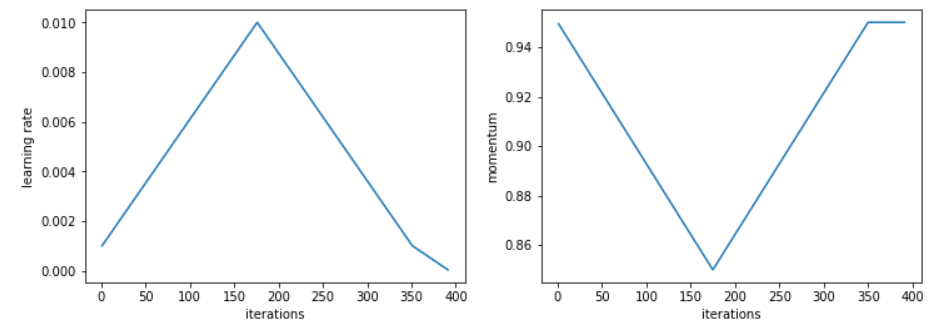Fig. 6 – Cyclical momentum has the shape that is akin to a horizontal flip of the one-cycle learning rate

## Learning Rate Finder

When using the Learning Rate Finder, it is important to use it with the same exact conditions as during training. For instance, we need to keep the batch sizes and weight decays the same as this will impact the optimal learning rate.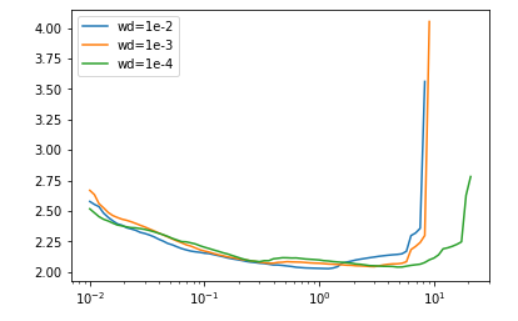Fig. 7 – Different weight decay values can result in different optimal learning rates

## Notes on the relationship between super-convergence to SGD and generalization

• In the paper Three Factors Influencing Minima in SGD by Jastrzębski et al, it was stated that higher levels of noise lead SGD to solutions with better generalization. The paper showed the ratio of learnign rate to the batch size, along with the variance of the gradient, controlled with the width of local minima found by SGD.
• In the paper Flat Minima by Hochreiter and Schmidhuber, wide, flat local minima produce solutions that generalize better than sharp minima. The super-convergence results align with the paper in the middle of training, yet small learning rate is necessary at the end of training, implying that the minima of the local minimum is narrow. This difference is yet to be solved.
• There are studies on the generalization gap between small and large mini-batches and between gradient noise, learning rate and batch size. Jastrzębski showed that SGD noise is proportional to learning rate, variance of loss gradients, divided by the batch size.
• In the paper On Large-Batch Training for Deep Learning: Generalization Gap and Sharp Minima by Keskar et al, it studies the generalization gap between small and large mini-batches, stating that small mini-batch sizes lead to wide, flat minima and large batch sizes lead to sharp minima. Paper also suggest to use a batch size warm start for the first few epochs before using a large batch size. This is ain to training with large gradient noise for a few epochs and then removing it. However, Leslie’s paper found this contradicting as his paper suggest to start training with little noise/regularization and let it increase (by increasing learning rate).
• In the paper Accurate, Large Minibatch SGD: Training ImageNet in 1 Hour by Goyal, they also suggest a gradual warm-up in learning rate and made a point to adjust the batch size along the way. If the network uses batch normalization, different mini-batch sizes leads to different statistics, which must be handled.

Reference:

1. https://sgugger.github.io/the-1cycle-policy.html

Papers:

1. A disciplined approach to neural network hyper-parameters: Part 1 — learning rate, batch size, momentum, and weight decay – Leslie N. Smith (https://arxiv.org/abs/1803.09820)
2. Super-Convergence: Very Fast Training of Neural Networks Using Large Learning Rates – Leslie N. Smith (https://arxiv.org/abs/1708.07120)
3. Curriculum Learning – Yoshua Bengio, Jérôme Louradour, Ronan Collobert, and Jason Weston (https://ronan.collobert.com/pub/matos/2009_curriculum_icml.pdf)
4. Simulated Annealing and Boltzmann Machines – Emile Aarts and Jan Korst (https://pdfs.semanticscholar.org/4569/26ae7135a4450859197506e87e75451dd6e1.pdf)